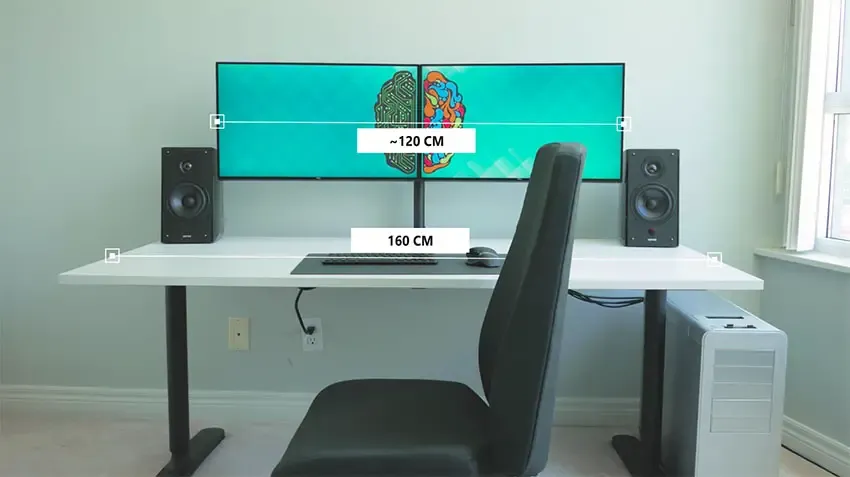# Programming - Can I run a Dual Monitor Setup? (C Code)Hello again! It's me drifter1 and I'm thinking of buying 2 new Monitors to make an Dual-Monitor Setup! It's boring to calculate again and again using mathematics (law of cosines) if 2 monitors with specific length (not diagonal monitor size, but horizontal) fit on an specific desk. So, I created a simple Code in C that uses the Law of Cosines and returns the number of degrees in which we have to put those 2 monitors so that they fit into the Desk. It also continues on calculating to check if we can have them stand out some cm's so that they become straight!

# C Code:

``#include <stdio.h>``
``#include <math.h>``

``int main(){``
``    int mon_length;``
``    int desk_length;``
`` ``
``    // get inputs``
``    printf("Monitor Length: ");``
``    scanf("%d", &mon_length);``
``    printf("Desk Length: ");``
``    scanf("%d", &desk_length);``
`` ``
``    // check if they fit straight directly``
``    if(mon_length*2 <= desk_length){``
`` printf("\nThey fit straight!\n");``
`` return 0;``
``    }``
`` ``
``    // calculate degrees with law of cosines``
``    double square_mon = pow(mon_length, 2);``
``    double square_desk = pow(desk_length, 2);``
``    double cosc = ``
``    (2 * square_mon - square_desk) / (2 * square_mon);``
``    double c = acos(cosc);``
`` ``
``    printf("\nAngle to put in:\n");``
``    printf("%.2f degrees\n\n", c*180/3.1415);``
`` ``
``    // calculate again with stand outs``
``    // until we again are straight``
``    int stand_out = 1;``
``    while(mon_length *2 > desk_length + stand_out){   ``
`` printf("With %d over desk:\n", stand_out);``
``             ``
`` double square_desk_over = pow(desk_length + stand_out, 2);``
`` double cosc_over = ``
``        (2 * square_mon - square_desk_over) / (2 * square_mon);``
`` double c_over = acos(cosc_over);``
`` printf("%.2f degrees\n\n", c_over*180/3.1415);``
``     ``
`` stand_out++;``
``    }``
`` ``
``    printf("After that they fit straight!\n");``
``}``

# Example Execution:

Suppose I want to buy 2 Monitors that are over 20 and under 30 inches diagonally. I do some research and I think of buying 2 IPS Monitors that are 24 or 27 inches. The 24" one has a horizontal length of 55cm and the 27" one a horizontal length of 61cm. My desk is 110cm long.

So, I come up with those results:

24" monitor:

Monitor Length: 55

Desk Length: 110

They fit straight!

27" monitor:

Monitor Length: 61

Desk Length: 110

Angle to put in:

128.75 degrees

And it continues on giving me values depending on the stand out, until we are over 11cm, where they fit straight!

So, then I can say, yeah the first ones fit and don't stand out when put straight, and the second ones will need some kind of angle that I will do getting either of the 2, and I can then buy depending not on size, but on budget and features!

Hope you enjoyed this post!

It is not something I thought of uploading, but I'm really thinking of buying 2 Monitors and so I went into creating this Code that let's me calculate if the monitors fit. I don't want to put them into an too big degree and I also don't have a lot of room of stand out at the sides of the desk. But the stand out with the 27" ones is not too big and so I might get those instead of 24" ones! Give me your thoughts on this :D

Until next time..Bye!

H2
H3
H4
3 columns
2 columns
1 column